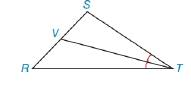Chapter 8.1, Problem 54EElementary Geometry For College St...

7th Edition
Alexander + 2 others
ISBN: 9781337614085

Solutions

Chapter
SectionElementary Geometry For College St...

7th Edition
Alexander + 2 others
ISBN: 9781337614085
Textbook Problem

T V ¯ bisects ∠ S T R of △ S T R . S T = 6 and T R = 9. If the area of △ S T R is 25  m 2 , find the area of △ S V T .To determine

To find:

The area of ΔSVT.

Explanation

“A median of a triangle separate it into two triangle of equal area.”

Calculation:

Given:

TV¯ bisects STR of ΔSTR.

ST=6 m

RT=9 m

ASTR=25 m2

TV¯ is bisect, the angle of ΔSTR.

(i.e.) TV¯ is the median of the line SR.

Since, A median of a triangle separate it into two triangle of equal area.

Let A1 and A2 are the areas of the triangle ΔSVT and ΔRVT.

Here, TV¯ bisects the ΔSTR into the two equal areas of triangles ΔSVT and ΔRVT

Still sussing out bartleby?

Check out a sample textbook solution.

See a sample solution

The Solution to Your Study Problems

Bartleby provides explanations to thousands of textbook problems written by our experts, many with advanced degrees!

Get Started

In Exercises 11-16, find the slope of the line that passes through each pair of points. 11. (4, 3) and (5, 8)

Applied Calculus for the Managerial, Life, and Social Sciences: A Brief Approach

Find the domain of the function. 37. F(p)=2p

Single Variable Calculus: Early Transcendentals

The graph at the right has equation:

Study Guide for Stewart's Multivariable Calculus, 8th

True or False: f(x) = 4x2 8x + 1 is increasing on (0, 1).

Study Guide for Stewart's Single Variable Calculus: Early Transcendentals, 8th